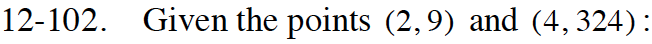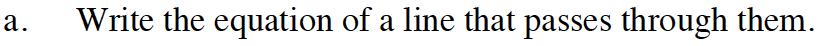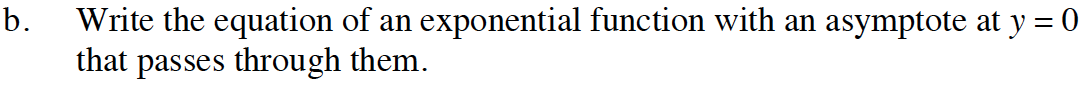Home > CCA2 > Chapter 12 > Lesson 12.2.1 > Problem12-102

12-102.
1. Given the points (2, 9) and (4, 324): Homework Help ✎

1. Write the equation of a line that passes through them.

2. Write the equation of an exponential function with an asymptote at y = 0 that passes through them.Use y = mx + b and the given points to solve for m and b.

9 = 2m + b
324 = 4m + bUse y = a(b)x and the given points to solve for a and b.

y = 0.25(6)x